# Selina Concise Mathematics Class 7 ICSE Solutions Chapter 6 Ratio and Proportion

## Selina Publishers Concise Mathematics Class 7 ICSE Solutions Chapter 6 Ratio and Proportion (Including Sharing in a Ratio)

Selina Publishers Concise Mathematics Class 7 ICSE Solutions Chapter 6 Ratio and Proportion (Including Sharing in a Ratio)

### Ratio and Proportion Exercise 6A – Selina Concise Mathematics Class 7 ICSE Solutions

Question 1.
Express each of the given ratio in its simplest form :Solution: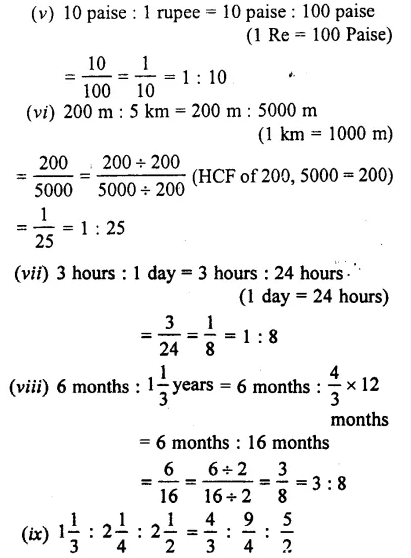Question 2.
Divide 64 cm long string into two parts in the ratio 5 : 3.
Solution:Question 3.
Rs. 720 is divided between x and y in the ratio 4:5. How many rupees will each get?
Solution:Question 4.
The angles of a triangle are in the ratio 3 :2 : 7. Find each angle.
Solution: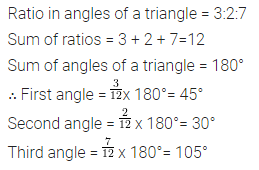Question 5.
A rectangular field is 100 m by 80 m. Find the ratio of
Solution:Question 6.
The sum of three numbers, whose ratios are 3 $$\frac { 1 }{ 3 }$$ : 4 $$\frac { 1 }{ 5 }$$ : 6 $$\frac { 1 }{ 8 }$$ is 4917.Find the numbers.
Solution: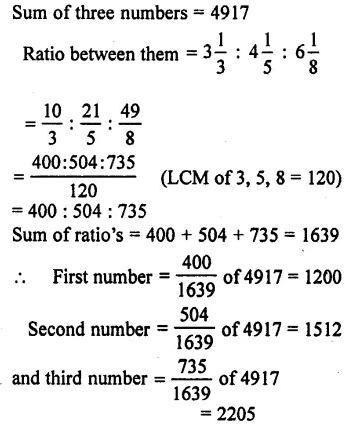Question 7.
The ratio between two quantities is 3 : the first is Rs. 810, find the second.
Solution:Question 8.
Two numbers are in the ratio 5 : 7. Their difference is 10. Find the numbers.
Solution:Question 9.
Two numbers are in the ratio 10 : 11. Their sum is 168. Find the numbers.
Solution:Question 10.
A line is divided in two parts in the ratio 2.5 : 1.3. If the smaller one is 35T cm, find the length of the line.
Solution:Question 11.
In a class, the ratio of boys to the girls is 7:8. What part of the whole class are girls.
Solution:Question 12.
The population of a town is ’ 50,000, out of which males are $$\frac { 1 }{ 3 }$$ of the whole population. Find the number of females. Also, find the ratio of the number of females to the whole population.
Solution:Question 13.
Ten gram of an alloy of metals A and B contains 7.5 gm of metal A and the rest is metal B. Find the ratio between :
(i) the weights of metals A and B in the alloy.
(ii) the weight of metal B and the weight of the alloy.
Solution:Question 14.
The ages of two boys A and B are 6 years 8 months and 7 years 4 months respectively. Divide Rs. 3,150 in the ratio of their ages.
Solution:Question 15.
Three persons start a business and spend Rs. 25,000; Rs. 15,000 atid Rs. 40,000 respectively. Find the share of each out of a profit of Rs. 14,400 in a year.
Solution: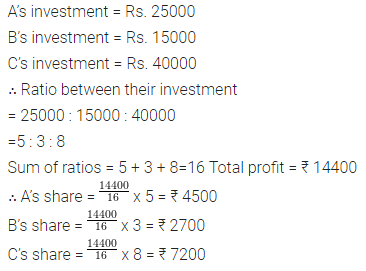Question 16.
A plot of land, 600 sq m in area, is divided between two persons such that the first person gets three-fifth of what the second gets. Find the share of each.
Solution:Question 17.
Two poles of different heights are standing vertically on a horizontal field. At a particular time, the ratio between the lengths of their shadows is 2 :3. If the height of the smaller pole is 7.5 m, find the height of the other pole.
Solution:Question 18.
Two numbers are in the ratio 4 : 7. If their L.C.M. is 168, find the numbers.
Solution:Question 19.
is divided between A and B in such a way that A gets half of B. Find :
(i) the ratio between the shares of A and B.
(ii) the share of A and the share of B.
Solution:Question 20.
The ratio between two numbers is 5 : 9. Find the numbers, if their H.C.F. is 16.
Solution:Question 21.
A bag contains ₹ 1,600 in the form of ₹10 and ₹20 notes. If the ratio between the numbers of ₹10 and ₹20 notes is 2 : 3; find the total number of notes in all.
Solution:Question 22.
The ratio between the prices of a scooter and a refrigerator is 4 : 1. If the scooter costs ₹45,000 more than the refrigerator, find the price of the refrigerator.
Solution: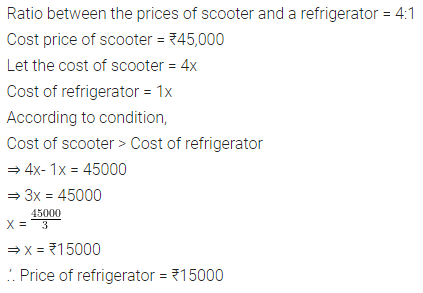### Ratio and Proportion Exercise 6B – Selina Concise Mathematics Class 7 ICSE Solutions

Question 1.
Check whether the following quantities form a proportion or not ?Solution:Question 2.
Find the fourth proportional ofSolution:Question 3.
Find the third proportional ofSolution: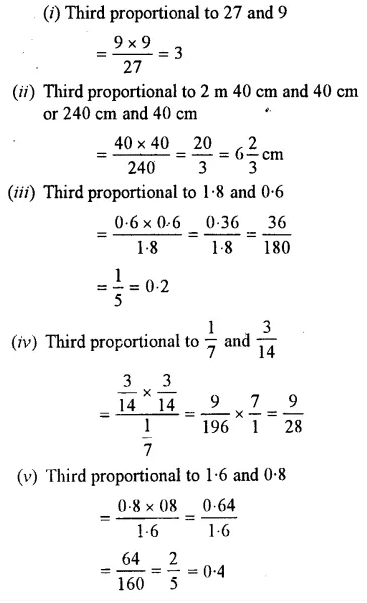Question 4.
Find the mean proportional betweenSolution:Question 5.Solution: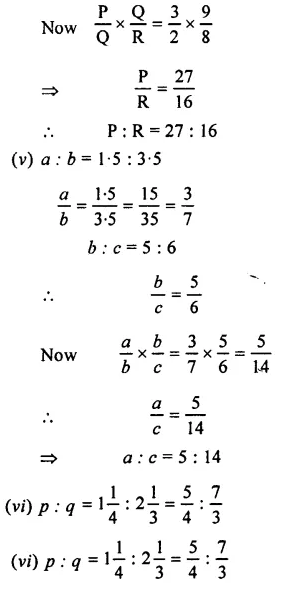Question 6.
If x: y – 5 :4 and 2 : x = 3 :8, find the value of y.
Solution: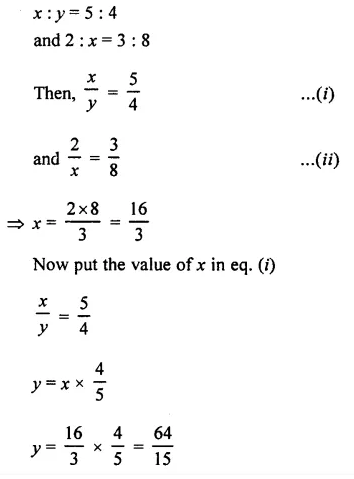Question 7.
Find the value of x, when 2.5 : 4 = x : 7.5.
Solution:Question 8.
Show that 2, 12 and 72 are in continued proportion.
Solution: